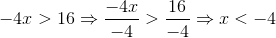# ACT Math : How to find the solution to an inequality with multiplication

## Example Questions

### Example Question #23 : Inequalities

Let x be a number such that x > 1 and x < 0. Let y be a number such that y > 0. Which of the following must be true?

(x/y) < 1

1 < xy < 1

x + y > 0

(x + y)2 > 1

(xy)2 > 1

(x/y) < 1

Explanation:

Let us see if we can find counterexamples for each choice so that we can eliminate it.

Let's look at the choice x+y > 0. We could let -0.75, and we could let y = 0.5, and this would satisfy the conditions for x and y. If x is -0.75 and y is 0.5, then x + y = -0.25 < 0, so this choice doesn't have to be true.

Let's then look at the choice -1 < xy < 1. If we let y be 10 and x be -0.5 then xy would be -5. This means that this choice isn't neccessarily true.

Let's look at the choice (xy)2 > 1. We could let x be -0.5 and we could let y be 1. Then xy would be -0.5, and (xy)2 would be 0.25 < 1. This means that this choice isn't always true.

Let's look at the choice (x + y)2 > 1. We could let y = 0.5 and x = -0.5. Then x + y would equal 0, and (x+y)2 = 0 < 1. We can eliminate this choice as well.

We suspect that (x/y) < 1 might be the answer, because we can contradict every other statement. However, let's see if we can prove that (x/y) has to be less than 1.

(x/y) < 1

We can multiply both sides by y, because y is positive, and this won't change the inequality sign. After multiplying both sides by y, we would have

x < y

Since y is always a positive number, and since x is always a negative number, this means that y will always be greater than x, so x < y must always be true.

### Example Question #24 : Inequalities

If the inequality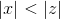is true, then which of the following must be true?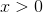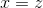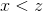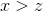Explanation:

Ifwe don't know anything for sure.could be less than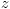, such as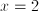and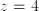.could also be greater than, such as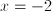and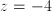.

In both of these cases,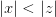.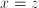cannot be true.

The other three choices COULD be true, but do not HAVE to be true.

### Example Question #25 : Inequalities

Which of the following is the solution set of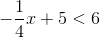?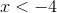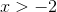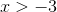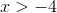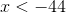Explanation: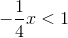Remember that when you multiply both sides of the inequality by a negative number you must switch the inequality sign around (less than becomes greater than; greater than becomes less than)

### Example Question #11 : Inequalities

If –1 < n < 1, all of the following could be true EXCEPT:

16n2 - 1 = 0

(n-1)2 > n

n2 < 2n

|n2 - 1| > 1

n2 < n

|n2 - 1| > 1

Explanation: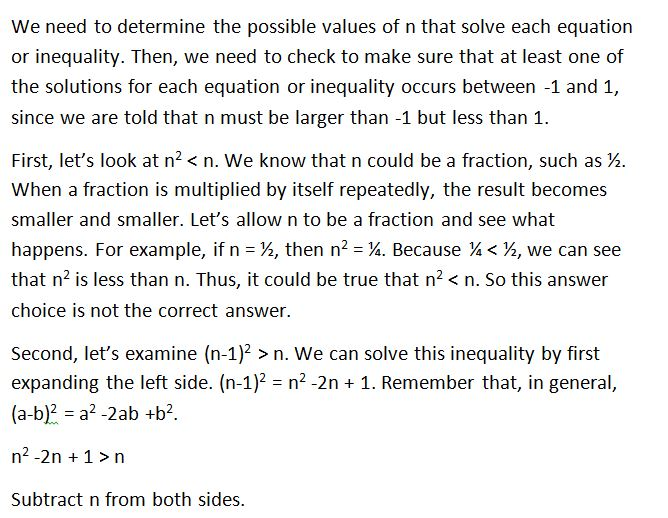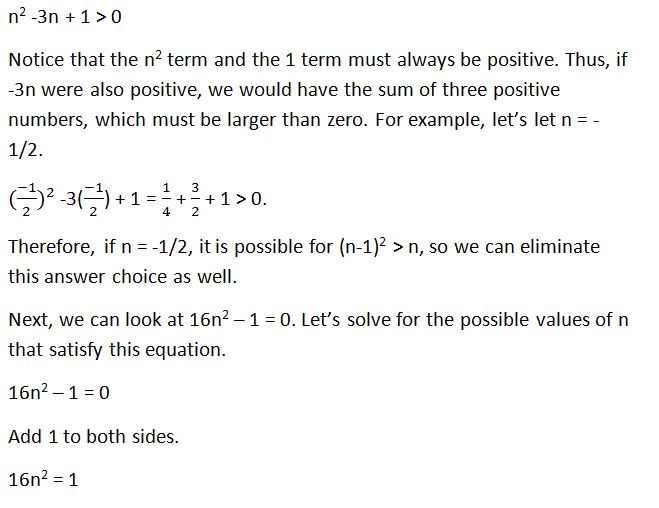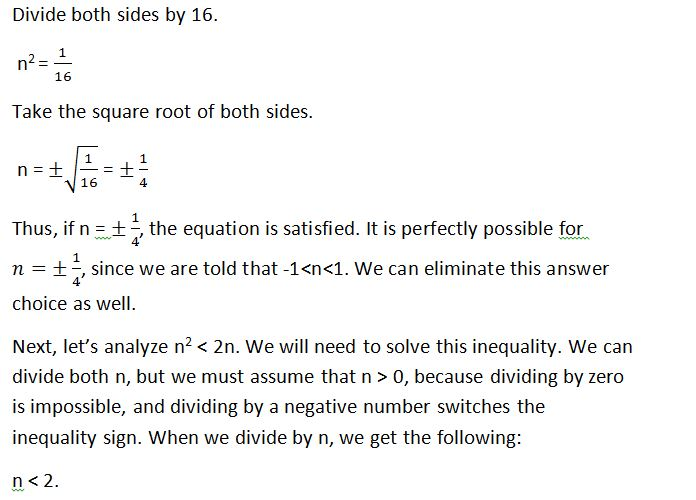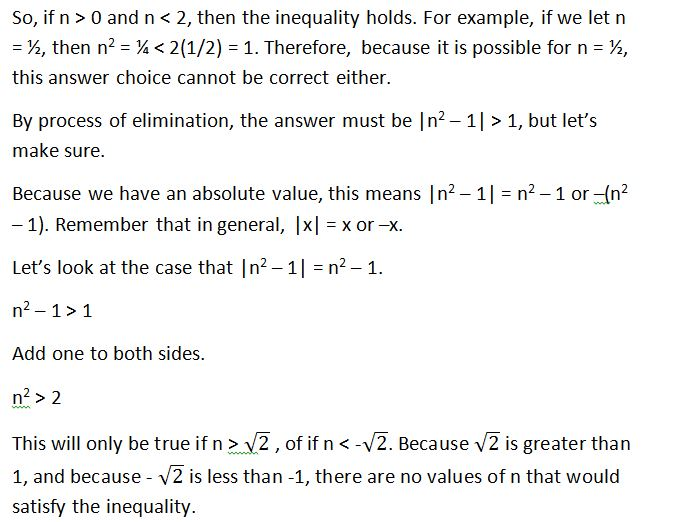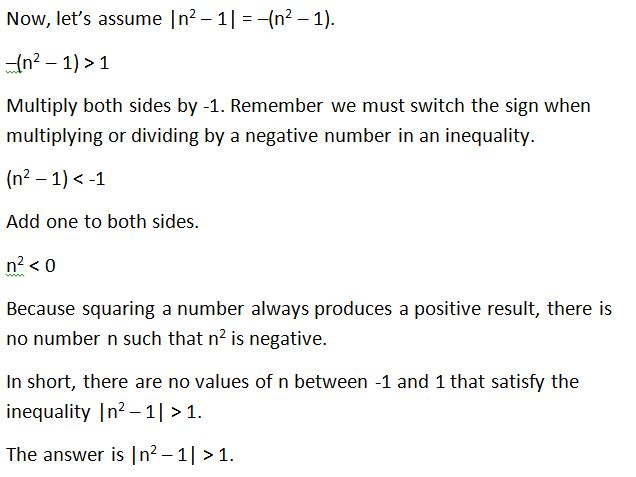### Example Question #2 : How To Find The Solution To An Inequality With Multiplication

(√(8) / -x ) <  2. Which of the following values could be x?

-4

-2

-1

All of the answers choices are valid.

-3

-1

Explanation:

The equation simplifies to x > -1.41. -1 is the answer.

### Example Question #221 : Algebra

Solve for x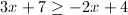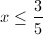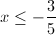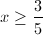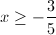Explanation: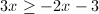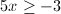### Example Question #4 : How To Find The Solution To An Inequality With Multiplication

We have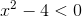, find the solution set for this inequality.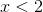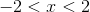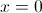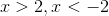Explanation: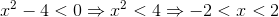### Example Question #151 : Algebra

Fill in the circle with either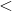,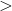, orsymbols: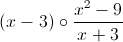for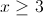.

The rational expression is undefined.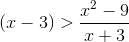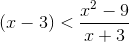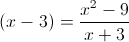None of the other answers are correct.Explanation:Let us simplify the second expression. We know that: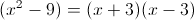So we can cancel out as follows: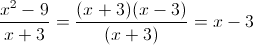### Example Question #1 : How To Find The Solution To An Inequality With Multiplication

Solve the following inequality: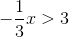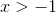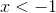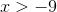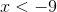Explanation:

To solve simply solve as though it is an equation.

The goal is to isolate the variable on one side with all other constants on the other side. Perform the opposite operation to manipulate the inequality.

However, remember that when dividing or multiplying by a negative number, you must flip the inequality sign.Multiply by -3, thus:### Example Question #2 : How To Find The Solution To An Inequality With Multiplication

Solve the following inequality: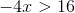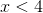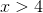Explanation:

To solve, simply treat it as an equation.

This means you want to isolate the variable on one side and move all other constants to the other side through opposite operation manipulation.

Remember, you only flip the inequality sign if you multiply or divide by a negative number.

Thus,